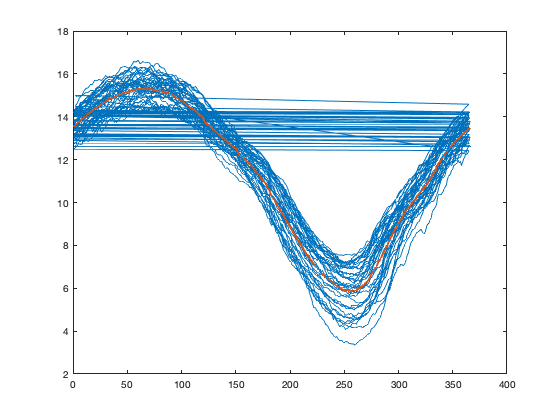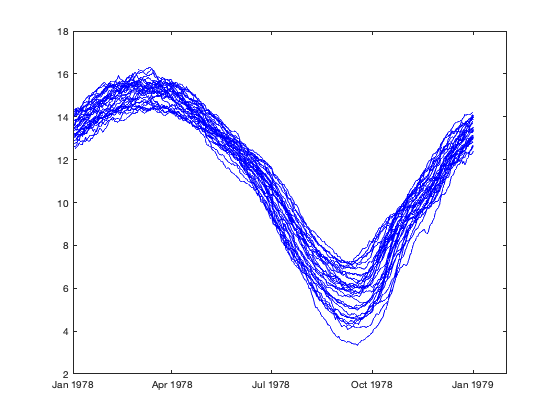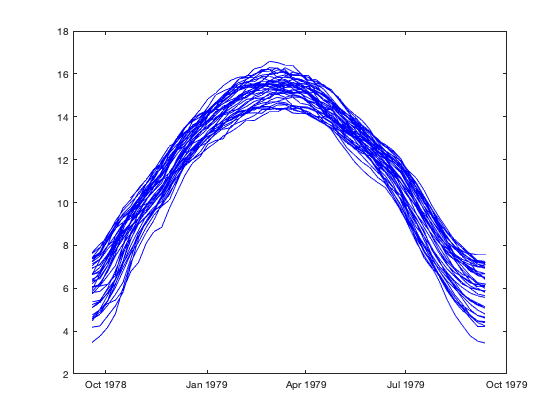# reshapetimeseries documentation

The reshapetimeseries function reshapes a vector of timeseries data onto a time-of-year x year grid, accounting for messiness associated with leap days or unevenly-spaced data.

Back to Climate Data Tools Contents

## Syntax

```[xg, yr] = reshapetimeseries(t, x)
[xg, yr] = reshapetimeseries(t, x, 'bin', nbin)
[xg, yr] = reshapetimeseries(t, x, 'bin', 'date')
[xg, yr] = reshapetimeseries(t, x, 'bin', 'month')
[xg, yr] = reshapetimeseries(t, x, 'func', @func)
[xg, yr] = reshapetimeseries(t, x, 'yrlim', yrlim)
[xg, yr] = reshapetimeseries(t, x, 'pivotdate, [mon day])```

## Description

[xg, yr] = reshapetimeseries(t, x) reshapes a timeseries x defined by time t onto a date-of-year by year grid. If the original timeseries is has higher than daily resolution, values are averaged for each date; for dates where the original timeseries included no data, NaNs are used. The resulting grid xg will be a 365 x n grid, where n is the number of unique years spanned by the the original timeseries; yr is a vector holding these year values.

[xg, yr] = reshapetimeseries(t, x, 'bin', nbin) reshapes the timeseries using nbin even time bins per year. Each bin will hold 365/nbin days worth of data in non-leap years and 366/nbin days worth of data in leap years.

[xg, yr] = reshapetimeseries(t, x, 'bin', 'date') [xg, yr] = reshapetimeseries(t, x, 'bin', 'month') These two special options for the 'bin' property allow for bins that align with calendar dates rather than evenly dividing each year. The 'date' option (the default option when no 'bin' value is specified) bins data from Feb 28-29 together, resulting in 365 bins per year that align with calendar dates, even in leap years. 'month' bins data by calendar month, resulting in 12 bins per year.

[xg, yr] = reshapetimeseries(t, x, 'func', @func) applies the function handle @func to data in a bin. @func should accept a vector of values and return a scalar. The default is to average data (i.e. @nanmean).

[xg, yr] = reshapetimeseries(t, x, 'yrlim', yrlim) returns a reshaped grid of data spanning the year limits in the 1 x 2 vector yrlim. This can be used to create an output dataset that spans more or fewer years than the input.

[xg, yr] = reshapetimeseries(t, x, 'pivotdate, [mon day]) set the pivot date where the dataset wraps from one year to the next. By default this is [1 1], indicating that each new column of the output xg starts on Jan 1 of the corresponding year in vector yr.

[xg, yr, tmid] = ... returns the additional output, tmid holding datetimes corresponding to the rows of xg. The year in this vector is set to the first non-leap year in the input dataset; this choice of year is relatively arbitrary. This output is intended primarily for plotting purposes, and the specific year can be adjusted by the user as needed.

## Example

When working with timeseries data, one often wants to analyze or plot things by time of year. For example, let's look at the sea ice extent example:

```load seaice_extent;

plot(t, extent_N);
```We can use doy to put this data on a day-of-year axis rather than datetimes:

```cla;
plot(doy(t), extent_N);
```But ugh, that wrapping from one year to the next prevents any quick and easy plotting. And we can calculate statistics across years by using splitapply or accumarray:

```[g, tdoy] = findgroups(floor(doy(t)));
iceavg = splitapply(@nanmean, extent_N, g);

hold on;
plot(tdoy, iceavg, 'linewidth', 2);
```But that syntax gets awfully clunky when you want to do more in-depth analysis. Both of these tasks would be a lot simpler if we could just reshape the data into matrix. The problem, of course, is those pesky leap days, always leading to uneven numbers of datapoints in different years and throwing off attempts to reshape. The reshapetimeseries function cleans up that messiness:

```[ice, yr, tmid] = reshapetimeseries(t, extent_N);

cla;
plot(tmid, ice, 'b')
hold on
```By default, it uses date bins, which makes sure to keep calendar dates aligned from one year to the next, and averages Feb 28-29 data in leap years. Monthly binning can also be achieved by setting the 'bin' input appropriately. (Note that the first year in this dataset started mid-October, which leads to the outlier-like point in the monthly averages).

```[icem, yr, tmidm] = reshapetimeseries(t, extent_N, 'bin', 'month');

plot(tmidm, icem, 'r')
```While Jan 1 is usually a good place to place the year-to-year wrap, sometimes your dataset may call for a different placement. For example, we can place the wrap near the low point of this timeries, in mid September. In this example, we choose 52 evenly-spaced bins per year, which is approximately a weekly average:

```[ice, yr, tmid] = reshapetimeseries(t, extent_N, ...
'pivotdate', [9 15], 'bin', 52);

cla
plot(tmid, ice, 'b')
hold on
```One thing to be aware of with this function is that it will add a NaN placeholder for any bins where no data existed in the original timeseries. Most of the time this will correspond to larger gaps in your timeseries, e.g. at the beginning or end if the timeseries doesn't start and end on the same calendar day. However, this can also occur if you choose a binning interval on a finer scale than your original data. For example, in this dataset, the early data is sampled every 2 days. If we try binning this on a daily basis, we end up with sparse data, staggered every other year:

```isearly = t < datetime(1988,1,1);
[ice, yr, tmid] = reshapetimeseries(t(isearly), extent_N(isearly));

tlbl = doy(tmid, 'decimalyear')- year(tmid(1));

figure;
imagesc(yr, tlbl, ice);
cmocean('ice');
xlabel('Year');
ylabel('Year fraction');
```In this case, we'd want to make sure we chose a larger interval when doing any real analysis:

```[ice, yr, tmid] = reshapetimeseries(t(isearly), extent_N(isearly), ...
'bin', floor(365/2));

figure;
imagesc(yr, tlbl, ice);